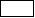# Boundaries of the study domain

## Introduction

To solve a problem by the finite element method, it is necessary:

• provide the boundaries of the field computation domain, i.e. define the external boundary surfaces of this domain,
• provide the boundary conditions, i.e. define the values of the state variable (the electric potential ) on the external boundaries of the field computation domain.

## Boundaries of the study domain with IB

In the majority of the cases, the technique of the infinite box (IB) is used. The boundary conditions are then assigned automatically by Flux on the infinite box (the electric potential at infinity is the potential of reference set by the user).

## Boundaries of the study domain without IB

If the technique of the infinite box (IB) is not used the boundary conditions on the border of the computation domain are set by the user by means of the regions known as non-material. The boundary conditions proposed are the following: normal electric field or tangential electric field.

In the absence of the boundary regions, the default boundary condition is applied. The electric field is tangent at the border.

## Symmetries and periodicities

If symmetries are assigned to the study domain, the user should provide the type of the symmetries. The electric field can be tangent or normal on the symmetry planes defined in the geometry context.

If periodicities are assigned to the study domain, the user should provide the type of the periodicities. Cyclic or anti-cyclic conditions can be imposed on the periodicity planes defined in the geometry context.

## Typical example

A typical example of problem treated as an Electro Static application is presented in the figure below.: Regions representing the main parts of the studied device (volume regions in 3D and surface regions in 2D): Regions representing the sources (face regions in 3D and line regions in 2D)